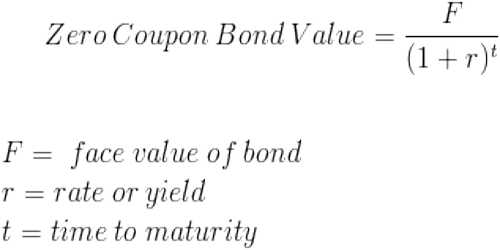# Zero Coupon Bond

A zero coupon bond (also called a discount bond or deep discount bond) is a bond bought at a price lower than its face value, with the face value repaid at tile time of maturity; it does not make periodic interest payments or has so-called coupons,” hence the term zero coupon bond. When the bond reaches maturity, its investor receives its par (or face) value. At maturity, it can then be redeemed at its face value allowing the bondholder to make a profit. Investors can purchase different kinds of zero coupon bonds in the secondary markets that have been issued from a variety of sources, including the U.S. Treasury, corporations, and state and local government entities.

The price of a zero-coupon bond can be calculated by using the following formula:

P = M / (1+r)n

where:

P = price

M = maturity value

r = investor’s required annual yield / 2

n = number of years until maturity x 2

Zero coupon bond prices are typically calculated using semi-annual periods (twice a year) because bonds that offer a coupon often pay interest twice a year.